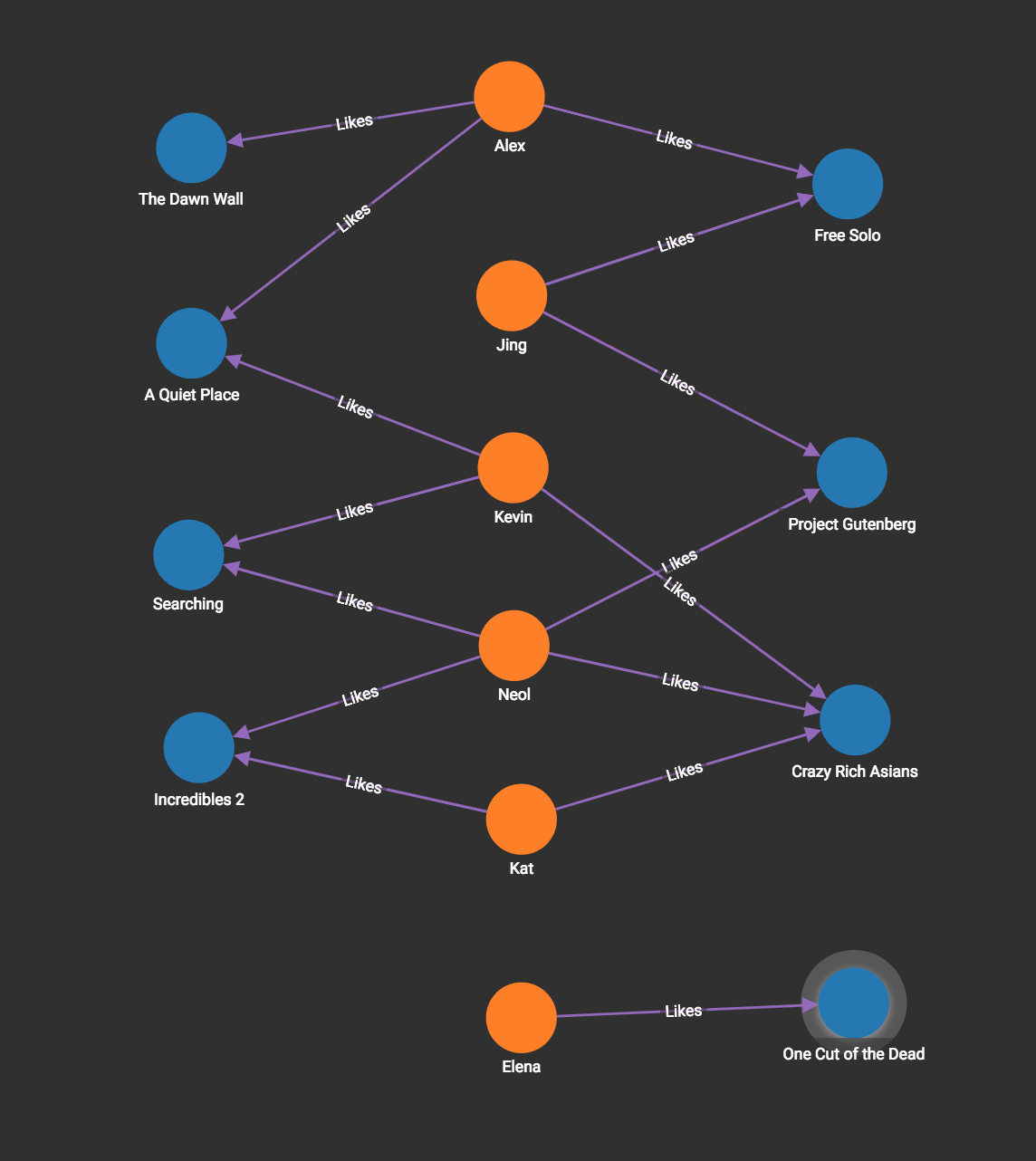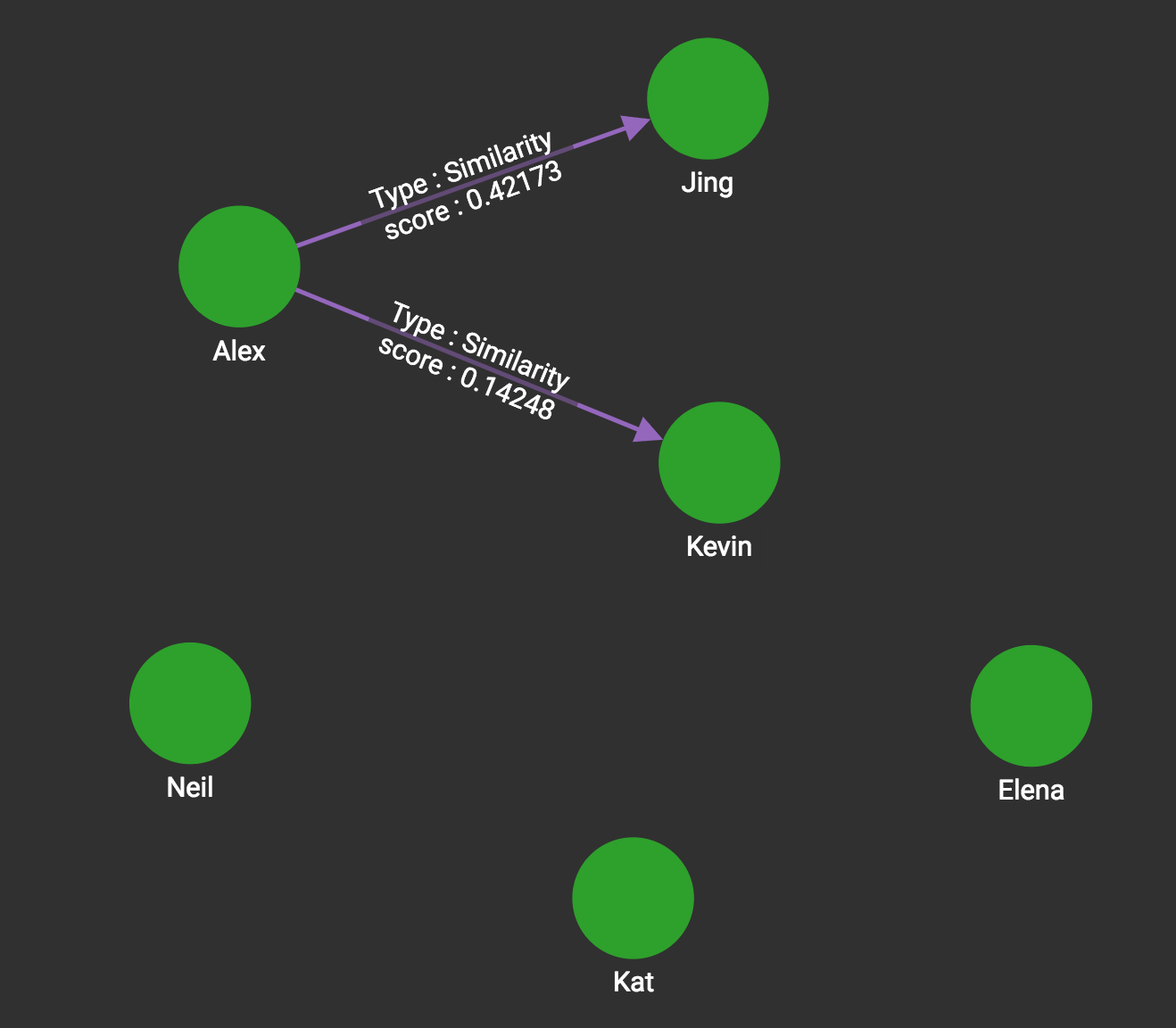# Cosine Similarity of Neighborhoods (Single-Source)

To compare two vertices by cosine similarity, the selected properties of each vertex are first represented as a vector. For example, a property vector for a Person vertex could have the elements age, height, and weight. Then the cosine function is applied to the two vectors.

The cosine similarity of two vectors A and B is defined as follows:

$cos(A,B)=\frac{A\cdot B}{||A||\cdot ||B||} = \frac{\sum_iA_i B_i}{\sqrt{\sum_iA^2_i}\sqrt{\sum_iB^2_i}}$

If A and B are identical, then cos(A, B) = 1. As expected for a cosine function, the value can also be negative or zero. In fact, cosine similarity is closely related to the Pearson correlation coefficient.

For this library function, the feature vector is the set of edge weights between the two vertices and their neighbors.

In the movie graph shown in the figure below, there are Person vertices and Movie vertices. Every person may give a rating to some of the movies. The rating score is stored on the Likes edge using the weight attribute. For example, in the graph below, Alex gives a rating of 10 to the movie "Free Solo".## Specifications

``````tg_cosine_nbor_ss (VERTEX source, SET<STRING> e_type, SET<STRING> re_type,
STRING weight, INT top_k, INT output_limit, BOOL print_accum = TRUE,
STRING file_path = "", STRING similarity_edge = "")
RETURNS (MapAccum<VERTEX, FLOAT>)``````
Characteristic Value

Result

The top K vertices in the graph that have the highest similarity scores, along with their scores.

The result is available in three forms:

• streamed out in JSON format

• written to a file in tabular format

• stored as a vertex attribute value

Input Parameters

• `VERTEX source`: Source vertex

• `SET<STRING> e_type`: Edge type to traverse

• `SET<STRING> re_type`: Reverse edge type to traverse

• `STRING weight:`The edge attribute to use as the weight of the edge

• `INT top_k`: Number of vertices

• `INT output_limit:`

• `BOOL print_accum`: If `true`, output JSON to standard output.

• `STRING filepath`: If provided, the output will be written to this file path in CSV format.

• `STRING similarity_edge`: If provided, the similarity score will be saved to this edge.

Result Size

`top_k`

Time Complexity

O(D^2), D = outdegree of vertex v

Graph Types

Undirected or directed edges, weighted edges

The output size is always K (if K ⇐ N), so the algorithm may arbitrarily choose to output one vertex over another if there are tied similarity scores.

## Example

Given one person’s name, this algorithm calculates the cosine similarity between this person and each other person where there is at one movie they have both rated.

In the previous example, if the source vertex is Alex, and `top_k` is set to 5, then we calculate the cosine similarity between him and two other persons, Jing and Kevin. The JSON output shows the top 5 similar vertices and their similarity score in descending order. The output limit is 5 persons, but we have only 2 qualified persons:

``````[
{
"@@result_topk": [
{
"vertex1": "Alex",
"vertex2": "Jing",
"score": 0.42173
},
{
"vertex1": "Alex",
"vertex2": "Kevin",
"score": 0.14248
}
]
}
]``````

The FILE version output is not necessarily in descending order. It looks like the following:

``````Vertex1,Vertex2,Similarity
Alex,Kevin,0.142484
Alex,Jing,0.421731``````

The ATTR version inserts an edge into the graph with the similarity score as an edge attribute whenever the score is larger than zero. The result looks like this: